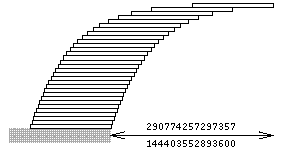A# book-stacking problemHow much of an overhang can be achieved by stacking books on a table? Assume each book is one unit long. To balance one book on a table, the center of gravity of the book must be somewhere over the table; to achieve the maximum overhang, the center of gravity should be just over the table's edge. The maximum overhang with one book is obviously 1/2 unit. For two books, the center of gravity of the first should be directly over the edge of the second, and the center of gravity of the stack of two books should be directly above the edge of the table. The center of gravity of the stack of two books is at the midpoint of the books' overlap, or (1 + 1/2)/2, which is 3/4 unit from the far end of the top book. It turns out that the overhangs are related to the harmonic numbers Hn, (see harmonic sequence) which are defined as 1 + 1/2 + 1/3 + ... + 1/n: the maximum overhang possible for n books is Hn/2. With four books, the overhang (1 + 1/2 + 1/3 + 1/4)/2. Thia exceeds 1, so that no part of the top book is directly over the table. With 31 books, the overhang is 2.0136 book lengths.